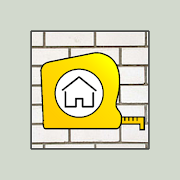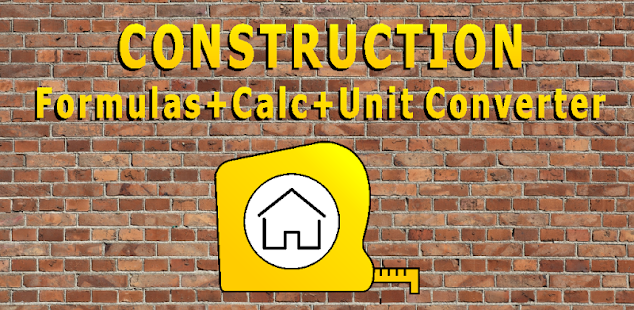# CONSTRUCTION calculatorEveryone
341In this application more than 150 formulas and tables is used. The range of calculations will be continuously expanded. You can submit your suggestions what calculations for the construction you need.

The application is defined by dynamic change of units. You can fill in one units of measurments and get the results in another selected units. There are about 300 units possible. Along with more complex calculations you will find a drawing.

Calculation results can be saved to make a template for the next calculation. Minimum data should be entered for the next calculation.Calculations in selected units needed for the construction.
The most popular shape, diagonal, perimeter, area, volume formulas and calculations.

Concreting Plastering
Masonry calculation
Tiles, paving tiles quantity
Filling the gap
Concrete composition
Gap calculation
Niches calculation
Stair-step calculation
Plasterboard
Calculation of the slope
Measurements
Calculation of proportions
Paint consumption calculation
Soil volume while digging a pond
Pipe diagonal cutting height
Electricity
Heating Ventilation
Quantity Area Volume Weight
Price Currency calculation
Areas volumes of geometric shapes
The time interval calculation
Rectangle
Right triangle
Circle
Triangle
Parallelogram
Trapezoid
Ellipse
Cone
Truncated Cone
Sphere
Prism
Pyramid
Truncated Pyramid
Wall area calculation
Area calculation
Length calculation
Water and air delivered by the heat
Heater selection
Fan selection
Voltage Current Power Resistance
Calculation of cord diameter (Long)
Material for wall structure
Material for partition
Material for ceilings
Decoration materials
Collapse

## Reviews

Review policy and info
2.7
341 total
5
4
3
2
1

## What's New

Correction of Errors
Collapse

Updated
October 27, 2020
Size
4.5M
Installs
100,000+
Current Version
2.7
Requires Android
4.4 and up
Content Rating
Everyone
Permissions
Offered By
Vizuali
Developer
Gvazdiku 6, 46317, Kaunas, Lietuva